﻿ A Unified Formula for The <i>n</i>th Derivative and The <i>n</i>th Anti-Derivative of the Bessel Function of Real Orders

### A Unified Formula for The nth Derivative and The nth Anti-Derivative of the Bessel Fun...

Mhenni M. Benghorbal

## A Unified Formula for The nth Derivative and The nth Anti-Derivative of the Bessel Function of Real Orders

Mhenni M. Benghorbal### Abstract

A complete solution to the problem of finding the nth derivative and the nth anti-derivative of elementary and special functions has been given. It deals with the problem of finding formulas for the nth derivative and the nth anti-derivative of elementary and special functions. We do not limit n to be an integer, it can be a real number. In general, the solution is given through unified formulas in terms of the Fox H-function and the Miejer G-function which, in many cases, can be simplified to less general functions. This, in turn, makes the first real use of these two special functions in the literature and shows the need of such functions. In this talk, we would like to present the idea on the Bessel function which is a well known special function. One of the key points in this work is that the approach does not depend on integration techniques. We adopt the classical definitions for generalization of differentiation and integration. Namely, the nth order of differentiation is found according to the Riemann-Liouville definitionwhere (k 1 < n < k) and. The generalized Cauchy n-fold integral is adopted for the nth order of integration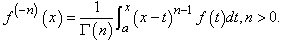• Mhenni M. Benghorbal. A Unified Formula for The nth Derivative and The nth Anti-Derivative of the Bessel Function of Real Orders. American Journal of Applied Mathematics and Statistics. Vol. 3, No. 3, 2015, pp 100-104. http://pubs.sciepub.com/ajams/3/3/3
• Benghorbal, Mhenni M.. "A Unified Formula for The nth Derivative and The nth Anti-Derivative of the Bessel Function of Real Orders." American Journal of Applied Mathematics and Statistics 3.3 (2015): 100-104.
• Benghorbal, M. M. (2015). A Unified Formula for The nth Derivative and The nth Anti-Derivative of the Bessel Function of Real Orders. American Journal of Applied Mathematics and Statistics, 3(3), 100-104.
• Benghorbal, Mhenni M.. "A Unified Formula for The nth Derivative and The nth Anti-Derivative of the Bessel Function of Real Orders." American Journal of Applied Mathematics and Statistics 3, no. 3 (2015): 100-104.

 Import into BibTeX Import into EndNote Import into RefMan Import into RefWorks

### 1. Introduction

The motivation of this work comes from the area of symbolic computation as well as the area of classical and fractional calculus. The idea is that: Given a function f in a variable x, can we or computer algebra systems (CAS) find a formula for the nth derivative, the nth anti-derivative, or both? This enhances the power of integration and differentiation of CAS. In Maple, the formula corresponds to invoking the command diff(f(x),x\$n) for the nth derivative and int(f(x), x\$n) for the nth anti-derivative. The latter command works only for n = 1.

The computation of fractional derivatives and fractional integrals involves evaluating some types of non elementary integrals. In fact, integration techniques do not work usually. This has led of thinking about different approaches to evaluate them. A series of papers has been established [1, 2, 3], to introduce different approaches to evaluating this type of integrals. This work is a continuation of this series. In addition to that a recent book  by the author.

### 2. The Bessel Function

The Bessel function of order(where0 is a constant ) is a solution of the Bessel differential equation of order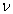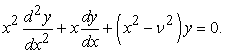(1)

The solution of the above differential equation can be found using the Frobenius series. The solution y(x), which represents the Bessel function, is given by the power series(2)

### 3. Riemann-Liouville Fractional Derivative Definition

The most widely known definition of the fractional derivative is The Riemann-Liouville definition (RLFD) [6, 7, 8]. It appears as a result of unification of the notions of integer-order integration and differentiation. The definition is given by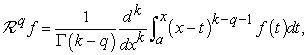(3)

where k 1 < q < k, k =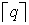, and f(x) is a function with a weak singularity over the interval of integration. If f(x) is continuous over the interval [a,x], then by letting q k, one gets f(k)(x).

3.1. The nth Derivative of (x a)m of Real Orders

We are interested in the fractional derivative (the nth derivative of real orders) of the function(4)

because of its latter use. Substituting (4) in (3) yields(5)

The above formula gives the nth derivative of real orders of the function (x a)m.

### 4. The Generalized Cauchy n-fold Integral Definition

The generalized Cauchy n-fold integral is a generalization of the Cauchy n-fold integral of integer order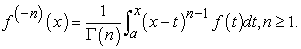Relaxing the condition on n in the above definition from integer n 1 to a real n > 0 does not affect the convergence of the integral. f(x) is a function with a weak singularity over the interval of integration.

4.1. The nth Anti-Derivative of (x a)m of Real Orders

Again, for our need of the nth anti-derivative of the function (x a)m, we give it in this section. It is the evaluation of the integral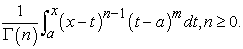Calculating the integral results in the desired formula(6)

### 5. The Mellin Transform

As a matter of fact, we need the mellin transform of the Bessel function, as it will be explained later, to find the desired unified formula. So, we introduce the Mellin transform and its inverse through the following two definitions. For more discussion of the Mellin transform; see .

Definition 1. : The Mellin transform  of a locally integrable function f(x) on (0,) is defined bywhere α and β are real constants. The stripis known as the strip of analyticity.

Definition 2. : The inverse Mellin transform  is given by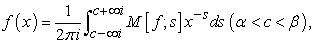where α and β are the same ones in the last definition.

Using the Mellin transform definition and evaluating the corresponding integral, an explicit expression for the Mellin transform of the Bessel function can be found(7)

where Γ(s) is the gamma function and is defined as(8)

### 6. The H-function

The H-function is a very general function that encompasses the most of special functions including the Meijer G-function and the generalized hypergeometric function; see [10, 11].

Notation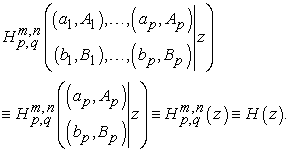Definition 3. The H-function is defined by the Mellin-Barnes integral .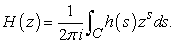where h(s) is given by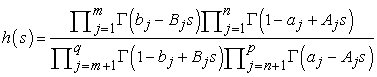(9)

For the above, we require that

(i) Aj and Bj are positive numbers.

(ii) aj and bj are complex numbers such that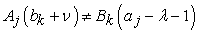forThat means the poles of Γ(bk Bks) for k = 1, , m and Γ(1 aj + Ajs) for j = 1, , n do not coincide.

(iii) The contour C separates the poles resulting from Γ(bk Bks) (1 k m) from those of Γ(1 aj + Ajs)(1 j n).

6.1. Existence Conditions for The H-function

For existence conditions of the H-function, we refer the reader to .

6.2. Properties of The H-function

The following are some properties of the H-function that are useful for our purpose (see ).

Property 1Property 2Property 3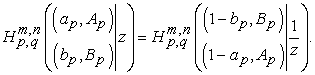### 7. Meijer G-function

The G-function is a special case of the H-function. A large number of special functions are special cases of this function. In this section we give some definitions of the function without any proofs. For a detailed discussion of the G-function, we refer the reader to .

NotationThese are the standard notations used in the literature. In the following definition an empty product is interpreted as unity and 0 m q, 0 n p. The Meijer G -function with the parameters a1, , ap and b1, , bq is defined as a Mellin-Barnes type integral as follows .

Definition 4.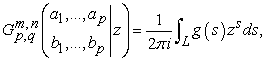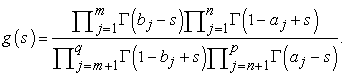It is clear that the Meijer G-function is a special case of the H-function and it is derived from the latter by letting Aj ’s and Bj ’s equal one.

7.1. The rth Derivative and The rth Anti-Derivative of The H-function

The closure property of the H-function under real orders of differentiation and integration makes it a very powerful tool for finding unified formulas for the nth derivative and the nth anti-derivative of elementary and special functions. In other words, one can express real order derivatives and integrals of an H-function in terms of a new H-function. This is a very nice property of the H-functions which is not possessed by other special functions. The following lemma illustrates the idea.

Lemma 1. The formula(10)

gives

(i) derivatives of arbitrary order if r > 0,

(ii) integrals of arbitrary order if r < 0,

of the H-function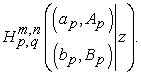Proof: We give a proof for part (i). The proof for part (ii) is similar to part(i) and only one needs to use formula (6) for fractional order integration. Recalling the definition of the H-function (9)where h(s) is given by (9). One can differentiate both sides of the above equation, provided the above integral converges uniformly for some z, using the formulagives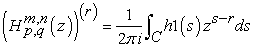where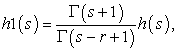h(s) is given by equation (9). Using the notation of the H-function, the above can be written as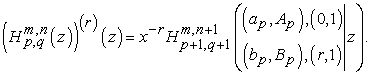Property (1) of the H-function simplifies the last equation to a more compact formIf C is taken as path (1), the existence condition for the above H-function isFor Part(ii), one needs formula (6)which is derived from the generalized Cauchy formula for the n-fold integral. The rest of the proof is exactly similar to part (i).

Formula (10) is very important for finding integer and arbitrary order symbolic derivatives and integrals of both elementary and special functions as long as they are representable in terms of the H-function.

### 8. A Unified Formula For The nth Derivative and The nth anti-Derivative of The Bessel Function of Real Orders

This section is devoted to introduce the complete solution to the problem of symbolic differentiations and integrations of the Bessel function of real orders. We lay down the theorem and its proof. The proof is given in steps that makes it clear and to the point.

Theorem 1. Let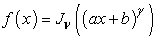be a Bessel function of order, where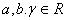, then the formula(11)

gives

(a) Derivatives of any order if n > 0,

(b) Anti-Derivatives of any order if n < 0,

(c) The original function if n = 0.

Proof: The first step in our proof requires taking the Mellin transform of our Bessel function which we have already found in section (5) and it is given by equation (7)(12)

Representing the Bessel function in terms of its inverse Mellin transform gives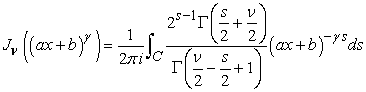where C is a suitable contour. Replacing s by s in the last equation yieldswith taking in consideration the change in the contour C. Using the H-function notation, we get the H-function representation of our Bessel function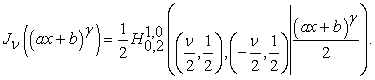By exploiting property (2) of the H-function, we can simplify the above H-function toApplying lemma (1), for real orders derivatives and anti-derivatives, to the above function gives a unified formula for the nth derivative and the nth anti-derivative of the Bessel function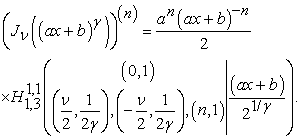(13)

A further simplification can be made to our unified formula by appealing to property (1)(14)

An existence condition, for the unified formula, can be found, and it is given by |(ax + b)| 0.

Example 1. : A unified formula for the nth derivative and the nth anti-derivative for the Bessel function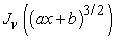can be found by substituting a = b, b = d, and= 3/2 in the unified formula (11). Simplifying the resulting H-function to a less general function, namely, the Meijer G-function, yields the unified formula(15)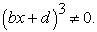The above G-functions reduce to the original function if n = 0. They give derivatives of any order if n > 0 and anti-derivatives of any order if n < 0.

### 9. Conclusion

The main achievement, in this work, can be summarized in the following statements. A complete solution to the problem of finding the nth derivative and the nth anti-derivative of the Bessel function of real orders is obtained. This can be considered as a big breakthrough in the area of classical and fractional calculus as well as the area of symbolic computation. The difficulty has been in the evaluation of non elementary integrals. The goal has been reached without appealing to integration techniques. The Fox H-function has been introduced, as a main tool, to give a complete solution to the problem in consideration. Another important fact is these unified formulas give a unification for the two classical definitions of generalized differentiation and integration. In the area of symbolic computation, these formulas enhance the power of computer algebra systems since they are plug in formulas. CAS such as Maple and Mathematica have already the Meijer G-function implemented. On the other hand, the Fox H-function has not been implemented yet.

### References

  Mhenni M. Benghorbal. A unified formula for arbitrary order symbolic derivatives and integrals of the power-exponential class. International Journal of Pure and Applied Mathematics, 37(3):349-365, 2007.In article  Mhenni M. Benghorbal. Unified formulas for integer and fractional order symbolic derivatives and integrals of the power-inverse trigonometric class I. International Journal of Pure and Applied Mathematics, 40(1):77-88, 2007.In article  Mhenni M. Benghorbal. A unified formula for the nth derivative and the nth-anti derivative of the power-logarithmic class. Proceedings of the International Conference of Computing in Engineering, Science and Information, California State University, Fullerton, California,IEEE, pages 31-34, 2009.In article  Mhenni Benghorbal. Fractional Differential Equations & Symbolic Derivatives and Integrals. Scholar’s Press, Germany, 2014.In article  M. Abramowitz and I.A. Stegun. Handbook of Mathematical Functions with Formulas, Graphs, and Mathematical Tables. Dover, 1970.In article  Keith B. Oldham and Jerome Spanier. The Fractional Calculus. Acadamic Press, 1974.In article  S.E. Samko, A.A. Kilbas, and O.I Marichev. Fractional Integrals and Derivatives, theory and applications. Gordon and Breach Science Publishers, 1996.In article  Igor Podlubny. Fractional Differential Equations. Academic Press, San Diego, California, 1999.In article  R.B. Paris and D. Kaminski. Asymptotics and Mellin-Barnes Integrals. Cambridge, 2001.In article View Article  Charles Fox. The G and H-functions as symmetrical fourier kernels. Trans. Amer. Math. Soc. 98, pages 395-429, 1961.In article  A.M. Mathai and R.K. Saxena. The H-function with Applications in Statistics and Other Disciplines. John Wiley and Sons, 1978.In article  A.M. Mathai. A Handbook of Generalized Special Functions for Statistical and Physical Sciences. Oxford Science Publications, 1993.In article PubMed